## Recommended Posts

Only sharing files and links for peoples that want to learn ..including mine self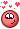Here its snippets(File) useful  for Tricks and Links for Video Tut  INC ...WITH  FILE.

uiHud.hiplc•6
•1

##### Share on other sites

Snippets that Only needs to Fine Adjust(for yours personal Pleasure) advertise For UI ...Need Help To Find  Sampler (POINTS) something like noise points that Dance..I know that I saw That here somewhere hmHave Fun.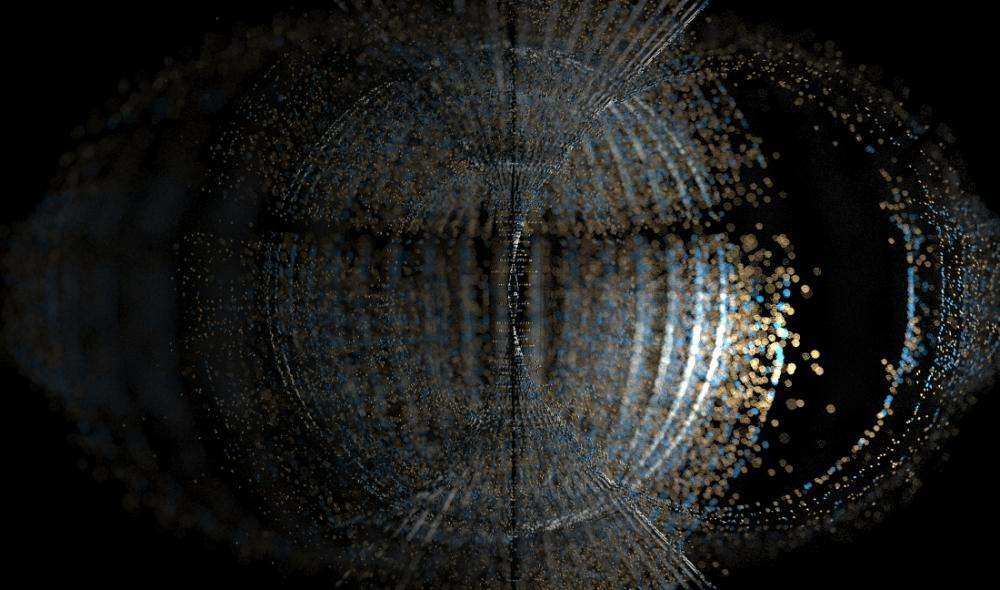Edited by Librarian
•1
•1

##### Share on other sites
•4

##### Share on other sites

More  Snippets .Have FunChop Iso Modulo PRIM CUTUIFUINOISEMODULOPRIM.hipnc

•1

##### Share on other sites

I made FUN(endless FUN) Have FUn..you can build( Cellular automata ..Game of Life) with this Setup @vinyvince  99.8%.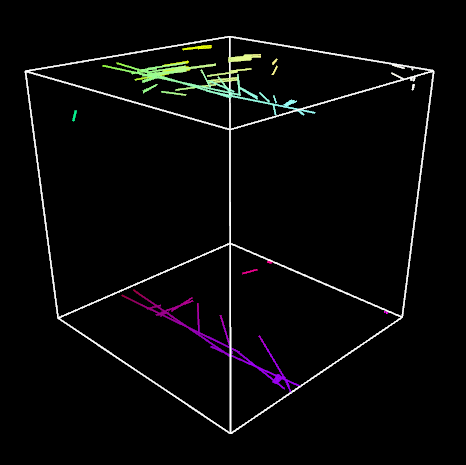POINTDANCEBOXCHOPSrleodforceUI.hiplc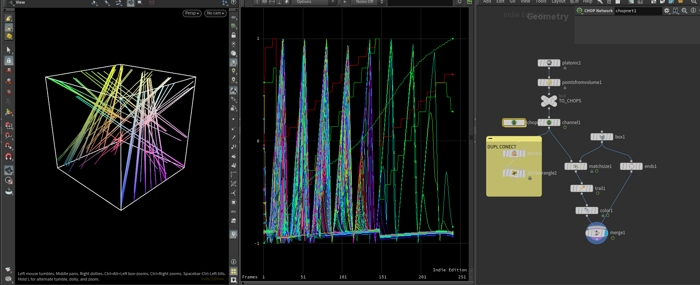•1
•1

##### Share on other sites

logarithm Functions and Trajectory I think maybe usefulFor this Topic

LOGTRAC.rar##### Share on other sites
•3
•1

##### Share on other sites

Cops Snippets and Convolve,

```float freq = .005;
vector pos = set (@IX *freq+20,@IY *freq,@TIME);
int seed = 123;
float f1, f2= 0;
wnoise(pos,seed,f1,f2);
float val= f1-abs(f2)-100;
@R= val;
@G= val;
@B= val;```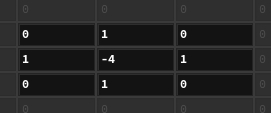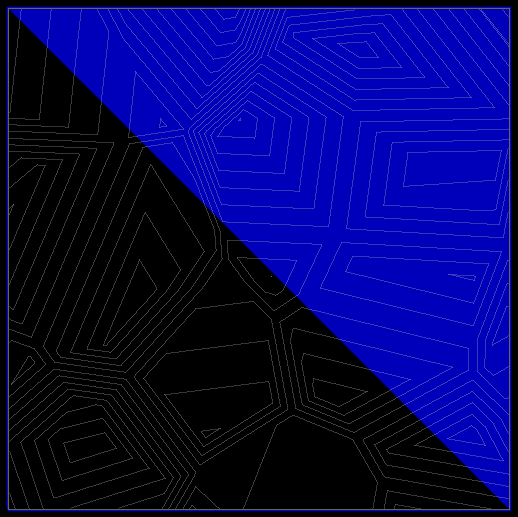##### Share on other sites

VEX CODES ONLY

VEXHYPERCAMERAPROJ.hiplc

Edited by Librarian

##### Share on other sites

Finding Beautiful patterns in Circle(grow connect Have Fun) endless possibility  ...Thanx to Asia Forums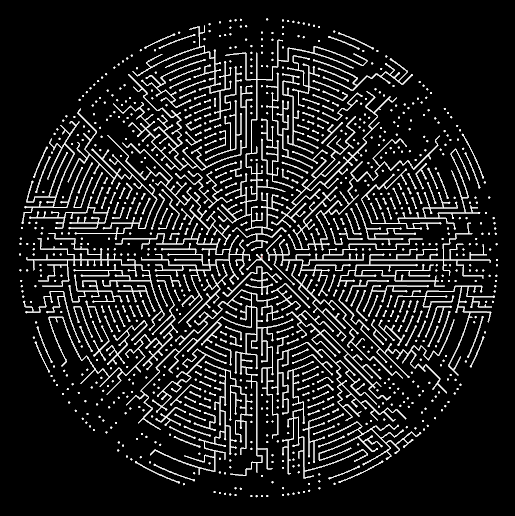BpatternsCircleGrow.hiplc

•1

##### Share on other sites

Grids Space 3

```int prim = addprim(0,"polyline");
float u;
int npt = chi("npt");
for (int i;i<npt;i++){
u = PI*2*float(i)/(npt-1);

vector pos = set(cos(@Time+u),sin(@Time+u),
sin((u+@Time)*2));

}```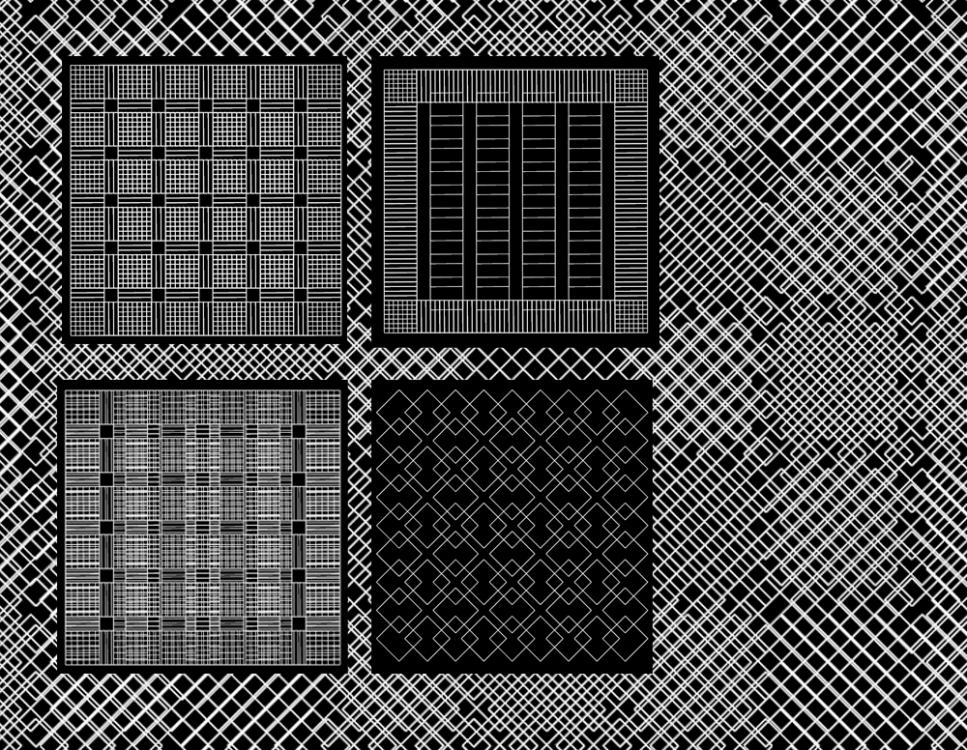##### Share on other sites

Patterns

```void rhombus (int n ;vector p;
float offset;
int bool;
float rolloff){

vector p0,p1,p2,p3;
if(bool){
p0={0,1,0};
p1={0.5,0.5,0}+(0.2+max(0,0.3*sin(offset)))*{1,1,0};
p2= {0.5,0.5,0}-(0.2+max(0,0.3*sin(offset)))*{1,1,0};
p3={1,0,0};
}else{
p0= 0;
p1={0.5,0.5,0}+(0.2+max(0,0.3*sin(offset)))*{1,-1,0};
p2= {0.5,0.5,0}-(0.2+max(0,0.3*sin(offset)))*{1,-1,0};
p3={1,1,0};
}

p0 +=p;
p1 +=p;
p2 +=p;
p3 +=p;

vector pos;
int pt;
float u;

int ni = 10 *n+1;
for(int i;i<ni;i++){
float u = i/float(ni-1);
u = smooth(0,1,u,rolloff);
pos = lerp(p1,p2,u);

}

}

vector pos;

for(int i;i<10*10;i++){

pos = set(i%10,i/10,0);
int bool = i%2;

if(i/10%2 == 0){
rhombus(0.4,pos,1.5,!bool,1.5*noise(@Time*i*0.1));
}else{
rhombus(2.1,pos,1.5,!bool,1.5*sin(@Time*i*0.1));
}
}

@width = 0.001;    ```

patt 2

```int octant [] = {};
for (int i ;i < 3;i++) octant[i]= floor(2*rand(i+@Time));

i[]@octant = octant;

int numelem = 9;
int dim = sqrt(numelem);
vector pos;
int pt;
for(int elemnum = 0;elemnum<numelem;elemnum++){

pos = set(elemnum%dim,elemnum/dim,0);

if(pos.x!= 1){
}else
if (octant[pos.y]){

}else{

removepoint(0,pt);

}

}

@width = 0.4;    ```

cir COL

```float r;
float theta;
vector p,v;
int n = 360*0.5;
int j, seed, prim;
float l;
float u;
int pt;

int t = min(n,1*@Frame);
while (j< 10){

r = rand(++seed);
l = max(0,350*rand(j)-5*@Time);
theta = t*4*PI/n;
p = r*set(cos(theta),sin(theta));
v = -set ( -p.y,p.x);
setpointattrib(0,"v",pt,v);
setpointattrib(0,"r",pt,r);

v = -set (-p.y,p.x);
setpointgroup(0,"group",pt,0);
if(theta > 3*PI)
setpointgroup(0,"group",pt,0);

if (r> 0.5){
j++;
for (int i;i<n;i++){
if(i<t && i>t-l){

u = fit(i,t-l+1,t-1,0,1);
theta = 4 *PI*i/n;
p = r*set(cos(theta),sin(theta));

setpointattrib (0,"Cd",pt,hsvtorgb(u+@Time,1,3*u));
setpointattrib (0,"Cd",pt,hsvtorgb(u,1,3*u));
setpointattrib (0,"width",pt,u*0.003);
setpointattrib (0,"r",pt,r);
v= -set (-p.y,p.x)*5;
setpointattrib (0,"v",pt,v);

}
}
}

}

```

##### Share on other sites

Have Fun
detail

```float r;
float theta;
vector p,v;
int n = 360*0.5;
int j, seed, prim;
float l;
float u;
int pt;

int t = min(n,1*@Frame);
while (j< 10){

r = rand(++seed);
l = max(0,350*rand(j)-50*@Time);
theta = t*4*PI/n;
p = r*set(cos(theta),sin(theta));
v = -set ( -p.y,p.x);
setpointattrib(0,"v",pt,v);
setpointattrib(0,"r",pt,r);

v = -set (-p.y,p.x);
setpointgroup(0,"group",pt,1);
if(theta > 6*PI)
setpointgroup(0,"group",pt,0);

if (r> 0.5){
j++;
for (int i;i<n;i++){
if(i<t && i>t-l){

u = fit(i,t-l+1,t-1,0,1);
theta = 4 *PI*i/n;
p = r*set(cos(theta),sin(theta));

setpointattrib (0,"Cd",pt,hsvtorgb(u+@Time,1,6*u));
setpointattrib (0,"Cd",pt,hsvtorgb(u,1,6*u));
setpointattrib (0,"width",pt,u*0.003);
setpointattrib (0,"r",pt,r);
v= -set (-p.y,p.x)*5;
setpointattrib (0,"v",pt,v);

}
}
}

}```

solver(emitter(detail) and trail)

```vector pos ;
vector v;
int ptnum;
int life ;
float r;
float maxangle;
float u;
int @age = 0;

foreach(int pt;expandpointgroup(1,"group")){

r = point(1,"r",pt);
v = point(1,"v",pt);

pos = point(1,"P",pt);
if(r>0.5){

for(int i;i<10*rand(pt);i++){

life = fit01(rand(r),0.4,1)*50+rand(i+pt);
setpointattrib(0,"life",ptnum,life);

v = sample_direction_cone(v,0.05,rand(i));
v = normalize(v)*float(rand(ptnum+pt));
setpointattrib(0,"v",ptnum,v);

}

}

}   ```

trail/points

```if (@age > @life) removepoint(0,@ptnum);

else{

@v += 0.05*onoise(@v*0.5)*@TimeInc;
@v += 0.5 *@TimeInc*float(@age)/@life*normalize(-@P);
//@v += -0.1*set(-@P.y,@P.x)*@TimeInc;
//@v = normalize(@v)*clamp(length(@v),0.1,1.2);
@P += @v *@TimeInc;
@age++;
float h = fit(@age,0,@life,1,0);
@Cd = hsvtorgb (h,1,1);

}
```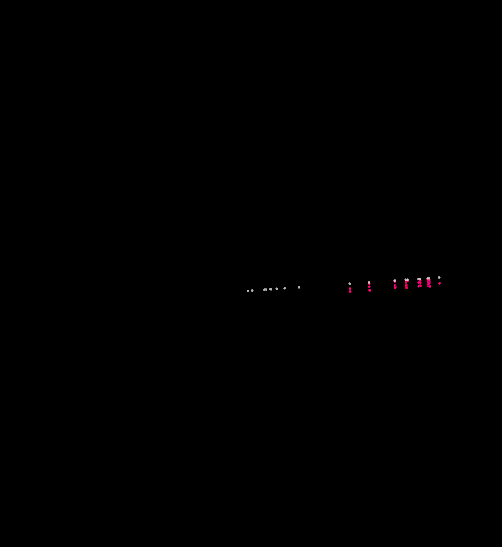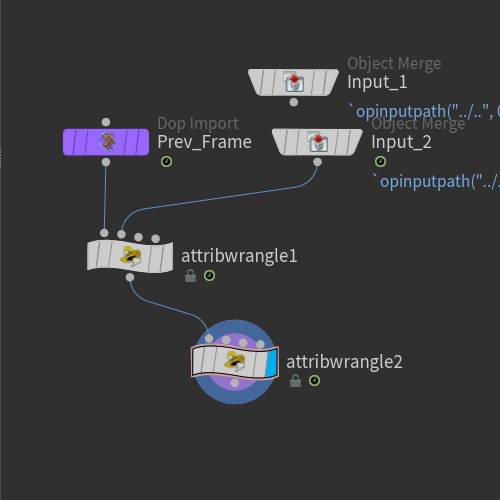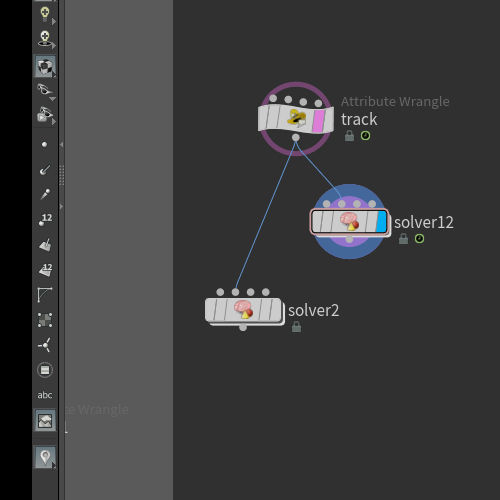##### Share on other sites
```string curve = "op:../curve4";
vector cur_uvw,next_uvw;
vector cur_center,next_center;
vector v,up,side,p;
setprimattrib(0,"Cd",prim,{1,0,0});
for (int i=1;i<@Frame +1;i++){
cur_uvw.x= fit(i,1,240,0,1);
next_uvw.x= fit(i+1,1,240,0,1);
cur_center = primuv(curve,"P",0,cur_uvw);
next_center=primuv(curve,"P",0,next_uvw);
v = next_center -cur_center;
if(i==1){
p = cur_center+0.2*rand(0);
}else{
p +=v;
v = normalize(v);
up = normalize(p-cur_center);
p += up *0.002;
side = normalize(cross(v,up));
p += side*0.05;

}

}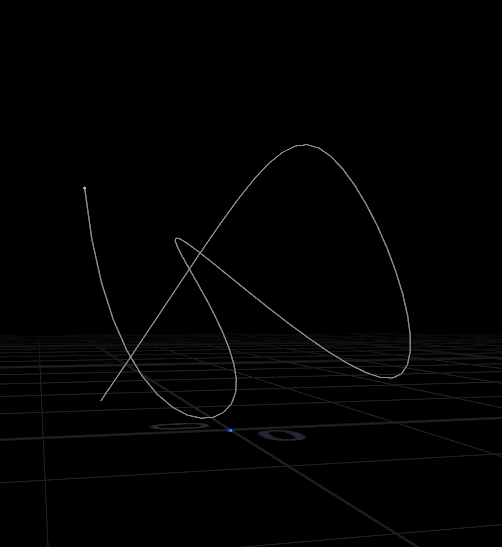##### Share on other sites
```for(int j;j<2;j++){
for(int i;i<n;i++){
u = -float(i)/(n-1);

pos.z = u;
t = clamp(-i+5*@Time,0,1);
pos.x = lerp(pos.x,0.3*pow(-1,j+1)*u*rand(10*u+50*(j+pow(-1,j))*0.01),t);
pos.x *= chramp("ramp",u);
if( i == n-1){

pos.x= pos.x+pow(-1,j)*0.5;
pos.z = 0;
}

pos = 0;

}

}```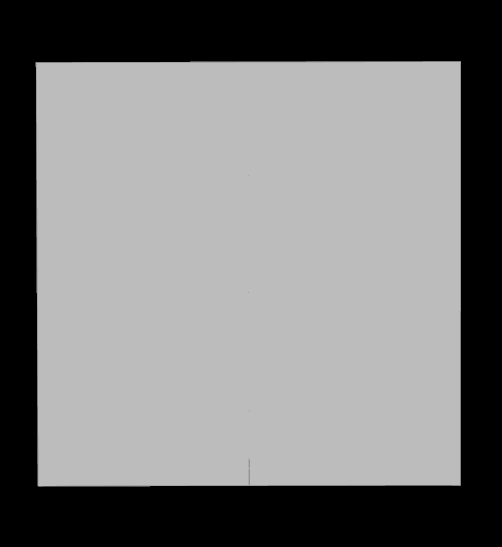##### Share on other sites
```int prim,pt;
float u;
for(int j;j <100 ;j++){
for(int i;i<100;i++){

setpointattrib(0,"u",pt,sin(PI*i/99.0));
}
}  ```

solver

```if (@Frame < 210 ){

float speed = ch("speed");
vector pos = {50,50} +30 *set(cos(speed*@Time),sin(speed*@Time));
vector deriv = set(-sin(speed*@Time),cos(speed*@Time));
float dist =distance(@P,pos);
float strength = ch("strength");
dist = fit(dist,0,1,strength,0);

@accel = deriv *dist;
} else{
@accel = 0;

}
v@vv = @accel;
@Cd = relpointbbox(0,@P) -length(@accel);

int near_pts[] = neighbours(0,@ptnum);
vector p0 = point(0,"P",near_pts);
vector p1 = point(0,"P",near_pts);

float length = ch("length")*fit01(@u,1,0.8);
if(distance(@P,p0)>1) @accel += (p0,@P)*length;
if(distance(@P,p1)>1) @accel += (p1,@P)*length;

if( len(neighbours(0,@ptnum))-1){
@v += @accel *@TimeInc ;
@P += @v *@TimeInc;
}

```## Create an account

Register a new account